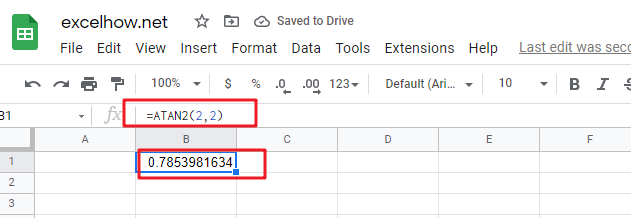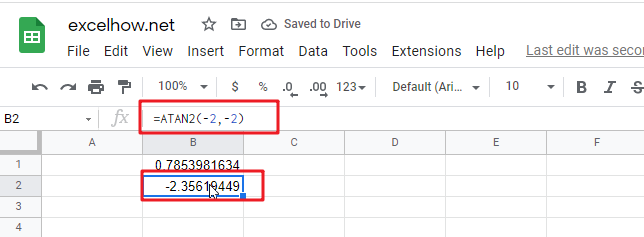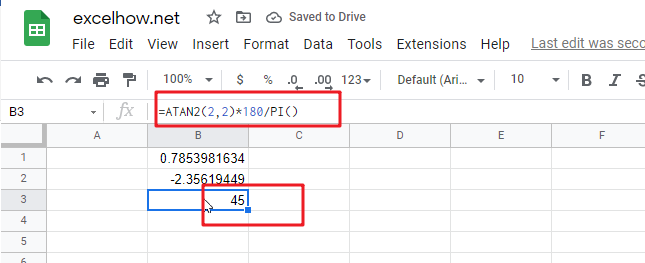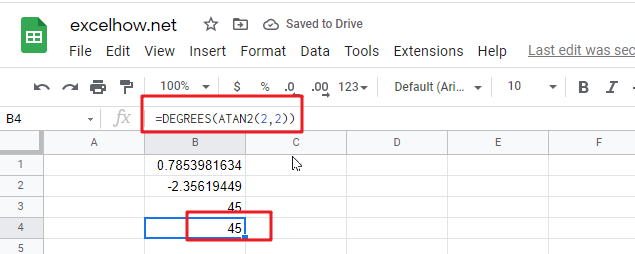# ExcelHow

This post will guide you how to use Google Sheets ATAN function with syntax and examples.

## Description

The Google Sheets ATAN function returns the arctangent, or inverse tangent, of the specified x- and y-coordinates. And you can use the ATAN2 function to calculate the arctangent of a given x and y coordinates. The returned value is an angle between –pi/2 and pi/2.

The purpose of ATAN function is to get arctangent from x- and y-coordinates in google sheets.

The ATAN function is a build-in function in Google Sheets and it is categorized as a MATH function.

## Syntax

The syntax of the ATAN2 function is as below:

= ATAN2 (x_num, y_num)

Where the ATAN2 function arguments are:

• x_num -This is a required argument. The x-coordinate of a point.
• Y_num – This is a required argument. The y-coordinate of a point.

Note:

• If the values of both x_num and y_num arguments are 0, the ATAN2 will return the #DIV/0! Error.
• The returned value of ATAN2(a,b) is equal to the formula ATAN2(b,a).
• If you want to express the arctangent in degrees, you just need to multiply the result by 180/PI() or you can use the DEGREES function.
• If the supplied number is not a numeric value, the ATAN function will get the #VALUE！ Error。
• If the returned result is a positive value, it indicates that it is a counterclockwise angle from the x-axis. If the returned result is a negative value, it indicates that it is a clockwise angle.

## Google Sheets ATAN Function Examples

The below examples will show you how to use google sheets ATAN2 Function to return the arctangent of x and y coordinates

1# get the arctangent of the x- and y-coordinates 2,2 in radians, enter the following formula in Cell B1.

=ATAN2(2,2)The returned result is 0.785398.

2# get the arctangent of the x- and y-coordinates -2,-2 in radians, -3*pi/4, using the following formula in Cell B2.

=ATAN2(-2,-2)This formula will return the value -2.35619.

3# get the arctangent of the x- and y-coordinates 2,2 in degrees, using the following formula in Cell B3.

=ATAN2(2,2)*180/PI()Or you can use the below formula to achieve the same result:

=DEGREES(ATAN2(2.2))